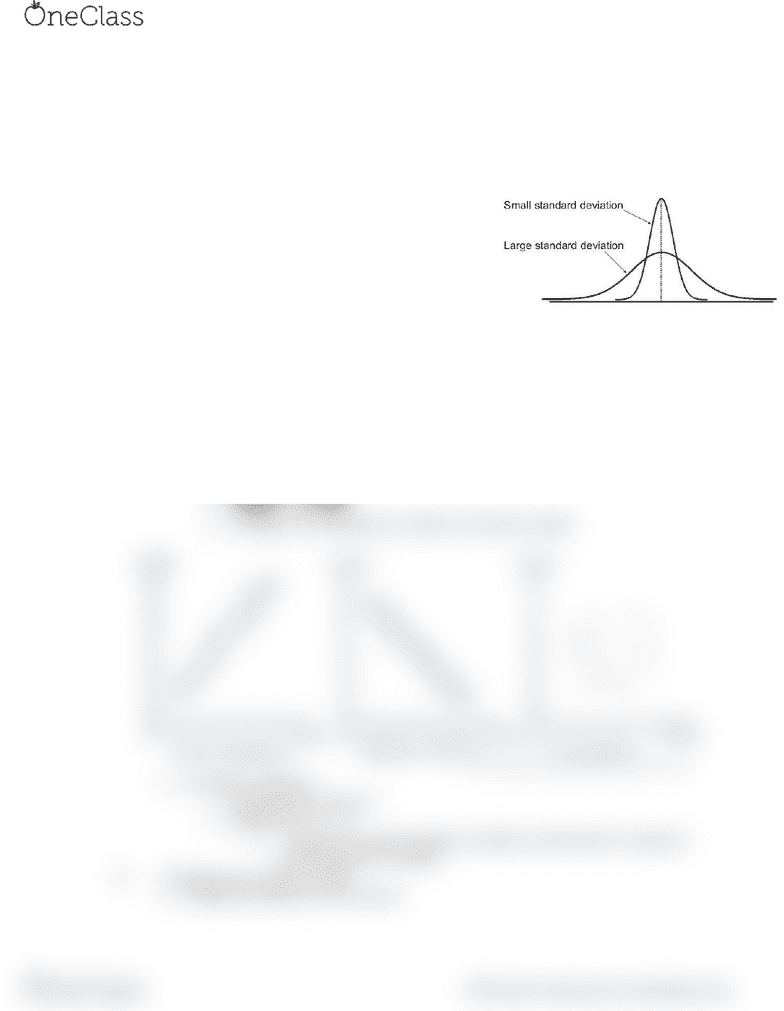# PSY 101 Lecture Notes - Lecture 3: Standard Deviation

45 views2 pages
School
Department
Course

For unlimited access to Class Notes, a Class+ subscription is required.Lecture 3 2-3-17
Quantifying Data and Statistical Analysis
I. Quantifying Data
a. Using a number to summarize variables
i. Variables any measurable characteristic that varies
b. This allows us to perform statistical analysis
II. Two methods of examining quantified data
a. Mean an arithmetic average of scores in a
distribution
b. Standard deviation the amount of
variability about the mean
i. A computed measure of how much
scores vary around the mean
III. Correlation
a. The degree of variability shared by two
variables
i. Association between two variables
b. Positive correlation
i. Example height and weight
ii. As one increases, the other also increases
c. Negative correlation
i. Example leisure time and work time
ii. As one increases, the other decreases
d. No correlation
i. Example SAT score
ii. A change in one variable has no effect on the other variable
e. Correlation coefficient
i. Denoted with the letter r
ii. Ranges from -1 < r < 1
1. -1 represents a perfect negative correlation, white positive 1 represents
a perfect positive correlation
IV. Comparing non-numeric variables
a. Categorical variables are non-numeric
https://fisherdong.wordpress.com/2011/03/25/positive-and-negative-correlation/
http://www.slideshare.net/nilanjanbhaumik9/measures-of-dispersion-43610696
find more resources at oneclass.com
find more resources at oneclass.com
Unlock document

This preview shows half of the first page of the document.
Unlock all 2 pages and 3 million more documents.

Already have an account? Log in

# Get access

Grade+
\$10 USD/m
Billed \$120 USD annually
Homework Help
Class Notes
Textbook Notes
40 Verified Answers
Study Guides
1 Booster Class
Class+
\$8 USD/m
Billed \$96 USD annually
Homework Help
Class Notes
Textbook Notes
30 Verified Answers
Study Guides
1 Booster Class